• 今天用matplotlib画方框，发现死活不上去，opencv一下子就上去了，但是jupyter用matplotlib居多，我这里分享一下我显示方框的代码： import numpy as np import matplotlib.pyplot as plt # 导入绘图包 from ...
今天用matplotlib画方框，发现死活画不上去，opencv一下子就画上去了，但是jupyter用matplotlib居多，我这里分享一下我显示方框的代码：
import numpy as np
import matplotlib.pyplot as plt # 导入绘图包
from PIL import Image
import matplotlib.patches as patches

img_path='/media/data/projects/EyesDetection/eyes/眼部打码/45度侧面像/A005969.JPG'
img = Image.open(img_path)
# print(img.size)
fig,ax = plt.subplots(1)
ax.imshow(img)
plt.figure("Image")
for point1 in points:
print(point1)
rect=patches.Rectangle(xy=(point1,point1),width=point1-point1,height=point1-point1,linewidth=2,fill=False,edgecolor='r')
ax.add_patch(rect)
我的目的是把voc 2007格式的数据显示出来

参考文献
.matplotlib：如何在图像上绘制一个矩形(matplotlib: how to draw a rectangle on image). https://www.it1352.com/896494.html
展开全文• 目录标题Matplotlib介绍什么是Matplotlib为什么要学习Matplotlib实现一个简单的Matplotlib画图认识Matplotlib图像结构拓展知识点：Matplotlib三层结构Matplotlib实操之折线图(plot)与基础绘图功能折线图绘制与保存...
 官网 https://matplotlib.org/

目录标题
Matplotlib介绍什么是Matplotlib为什么要学习Matplotlib实现一个简单的Matplotlib画图认识Matplotlib图像结构拓展知识点：Matplotlib三层结构

Matplotlib实操之折线图(plot)与基础绘图功能折线图绘制与保存图片实际应用之显示图片实际应用之保存图片
完善原始折线图1(辅助显示层)提前加入的代码（中文乱码，图像风格，字体）1. 准备数据并画出初始折线图2. 添加自定义x,y刻度3. 中文显示问题解决4. 添加网格显示5. 添加描述信息
完善原始折线图2(图像层)1 多次plot2 设置图形风格两次完善的总结代码多个坐标系显示-plt.subplots(面向对象的画图方法)
小结

Matplotlib介绍
什么是Matplotlib
是专门用于开发2D图表(包括3D图表)使用起来及其简单以渐进、交互式方式实现数据可视化
为什么要学习Matplotlib
可视化是在整个数据挖掘的关键辅助工具，可以清晰的理解数据，从而调整我们的分析方法。
能将数据进行可视化,更直观的呈现使数据更加客观、更具说服力
例如下面两个图为数字展示和图形展示：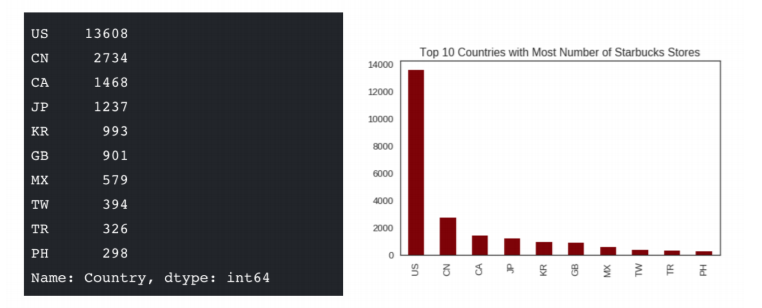实现一个简单的Matplotlib画图
import matplotlib.pyplot as plt
plt.figure(figsize=(20,8),dpi = 100)
plt.plot([1,2,3],[4,5,6])
plt.show()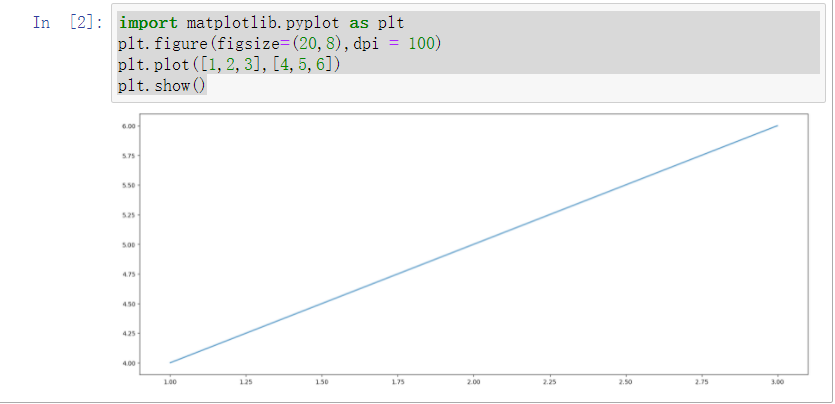认识Matplotlib图像结构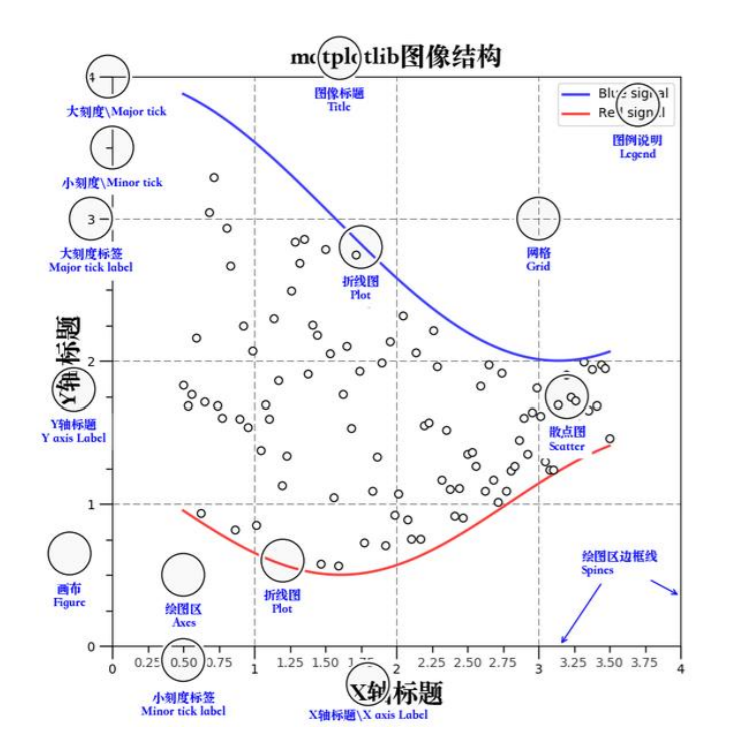拓展知识点：Matplotlib三层结构
容器层 容器层主要由Canvas、Figure、Axes组成。 Canvas是位于最底层的系统层，在绘图的过程中充当画板的角色，即放置画布(Figure)的工具。 Figure是Canvas上方的第一层，也是需要用户来操作的应用层的第一层，在绘图的过程中充当画布的角 色。 Axes是应用层的第二层，在绘图的过程中相当于画布上的绘图区的角色。
Figure:指整个图形(可以通过plt.figure()设置画布的大小和分辨率等)Axes(坐标系):数据的绘图区域Axis(坐标轴)：坐标系中的一条轴，包含大小限制、刻度和刻度标签
特点为：
一个figure(画布)可以包含多个axes(坐标系/绘图区)，但是一个axes只能属于一个figure。一个axes(坐标系/绘图区)可以包含多个axis(坐标轴)，包含两个即为2d坐标系，3个即为3d坐标系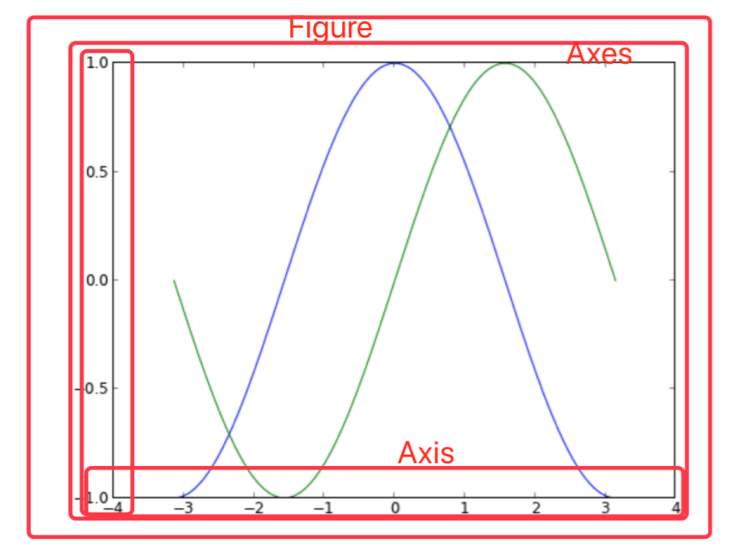辅助显示层
辅助显示层为Axes(绘图区)内的除了根据数据绘制出的图像以外的内容，主要包括Axes外观 (facecolor)、边框线(spines)、坐标轴(axis)、坐标轴名称(axis label)、坐标轴刻度(tick)、坐标轴刻度标 签(tick label)、网格线(grid)、图例(legend)、标题(title)等内容。该层的设置可使图像显示更加直观更加容易被用户理解，但又不会对图像产生实质的影响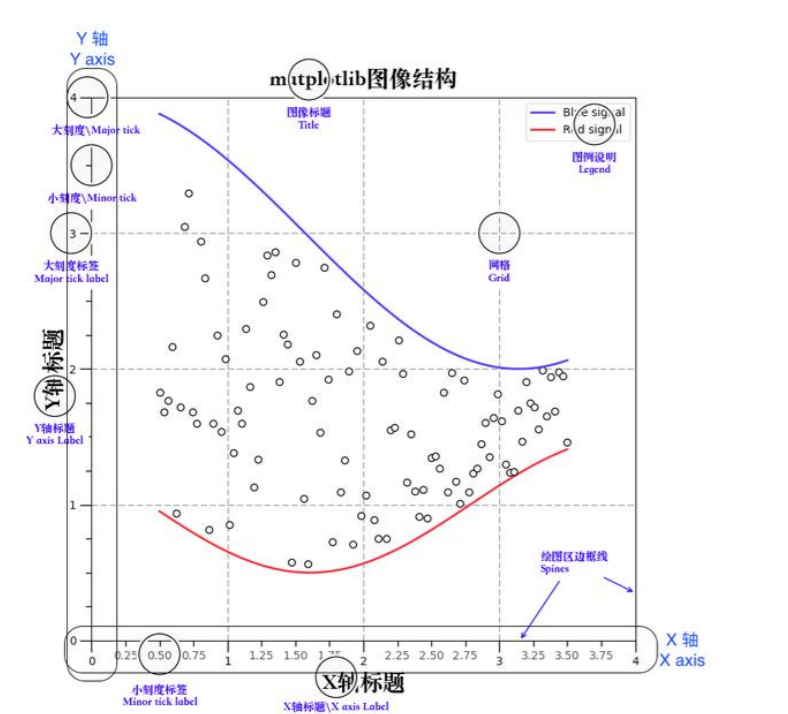图像层 图像层指Axes内通过plot、scatter、bar、histogram、pie等函数根据数据绘制出的图像。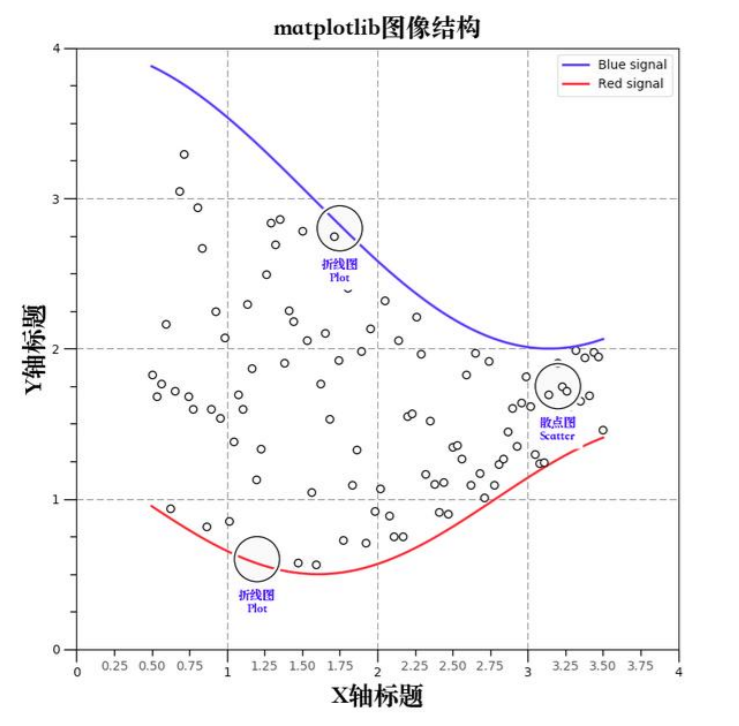总结：
Canvas（画板）位于最底层，用户一般接触不到Figure（画布）建立在Canvas之上Axes（绘图区）建立在Figure之上坐标轴（axis）、图例（legend）等辅助显示层以及图像层都是建立在Axes之上
Matplotlib实操之折线图(plot)与基础绘图功能
折线图绘制与保存图片
matplotlib.pytplot包含了一系列类似于matlab的画图函数。 它的函数作用于当前图形(figure)的当前 坐标系(axes)。  设置画布属性与图片保存 注意：plt.show()会释放figure资源，如果在显示图像之后保存图片将只能保存空图片
plt.figure(figsize=(), dpi=)
# figsize:指定图的长宽
# dpi:图像的清晰度
# 返回fig对象
plt.savefig(path)

实际应用之显示图片
import matplotlib.pyplot as plt

# 1）创建画布(容器层)
plt.figure(figsize=(10, 10),dpi=80)
# 2）绘制折线图(图像层)
plt.plot([1, 2, 3, 4, 5, 6 ,7], [17, 17, 18, 15, 11, 11, 13])
# 3）显示图像
plt.show()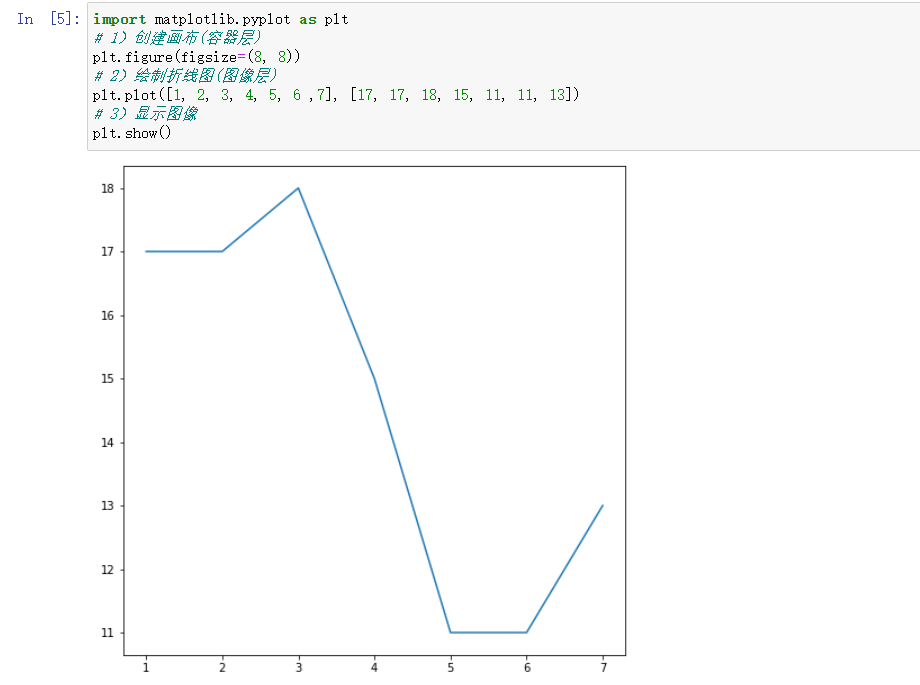实际应用之保存图片
注意：plt.show()会释放figure资源，如果在显示图像之后保存图片将只能保存空图片
### 实际应用之显示图片### 实际应用之保存图片
import matplotlib.pyplot as plt
# 1）创建画布(容器层)
plt.figure(figsize=(8, 8))
# 2）绘制折线图(图像层)
plt.plot([1, 2, 3, 4, 5, 6 ,7], [17, 17, 18, 15, 11, 11, 13])
# 3）保存图片到指定路径
plt.savefig('test.png')

完善原始折线图1(辅助显示层)
提前加入的代码（中文乱码，图像风格，字体）
plt.style.use('seaborn')  # 改变图像风格
plt.rcParams['font.family'] = ['Arial Unicode MS', 'Microsoft Yahei', 'SimHei', 'sans-serif']  # 解决中文乱码
plt.rcParams['axes.unicode_minus'] = False  # simhei黑体字 负号乱码 解决

1. 准备数据并画出初始折线图
# 完善原始折线图1(辅助显示层)

# 准备x, y坐标的数据
x = range(60)
y_shanghai = [random.uniform(15,18) for i in x]

# 1 创建绘布
plt.figure(figsize=(20,8),dpi=80)

# 2 绘制折线图
plt.plot(x,y_shanghai)

# 3 显示图像
plt.show()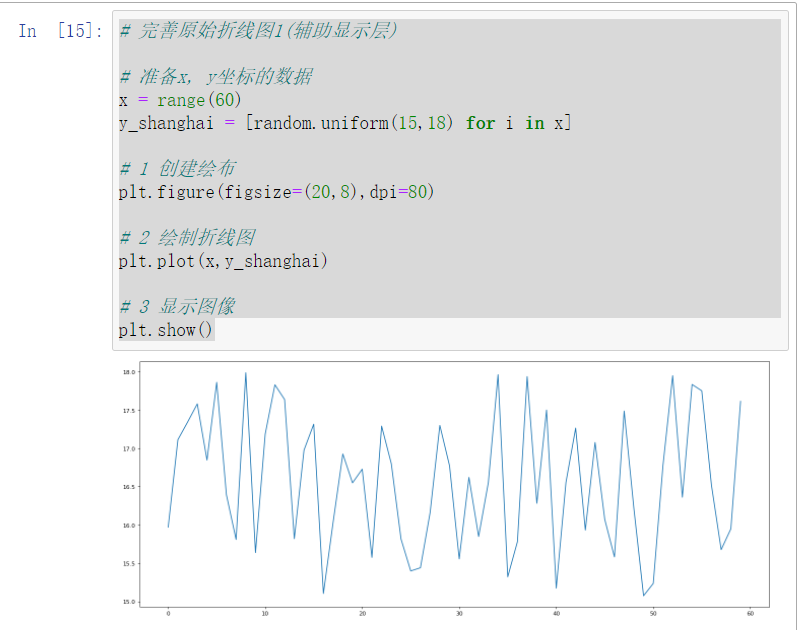2. 添加自定义x,y刻度
plt.xticks(x, **kwargs) x:要显示的刻度值plt.yticks(y, **kwargs) y:要显示的刻度值
# 完善原始折线图1(辅助显示层)
# 2 添加自定义x,y刻度

# 准备x, y坐标的数据
x = range(60)
y_shanghai = [random.uniform(15,18) for i in x]

# 1 创建绘布
plt.figure(figsize=(20,8),dpi=80)

# 2 绘制折线图
plt.plot(x,y_shanghai)
# 构造x轴刻度标签
x_ticks_label = ['11点{}分'.format(i) for i in x]
# 构造y轴刻度
y_ticks_label = range(40)
# 修改x,y轴坐标的刻度显示
plt.xticks(x[::5],x_ticks_label[::5])
plt.yticks(y_ticks_label[::5])

# 3 显示图像
plt.show()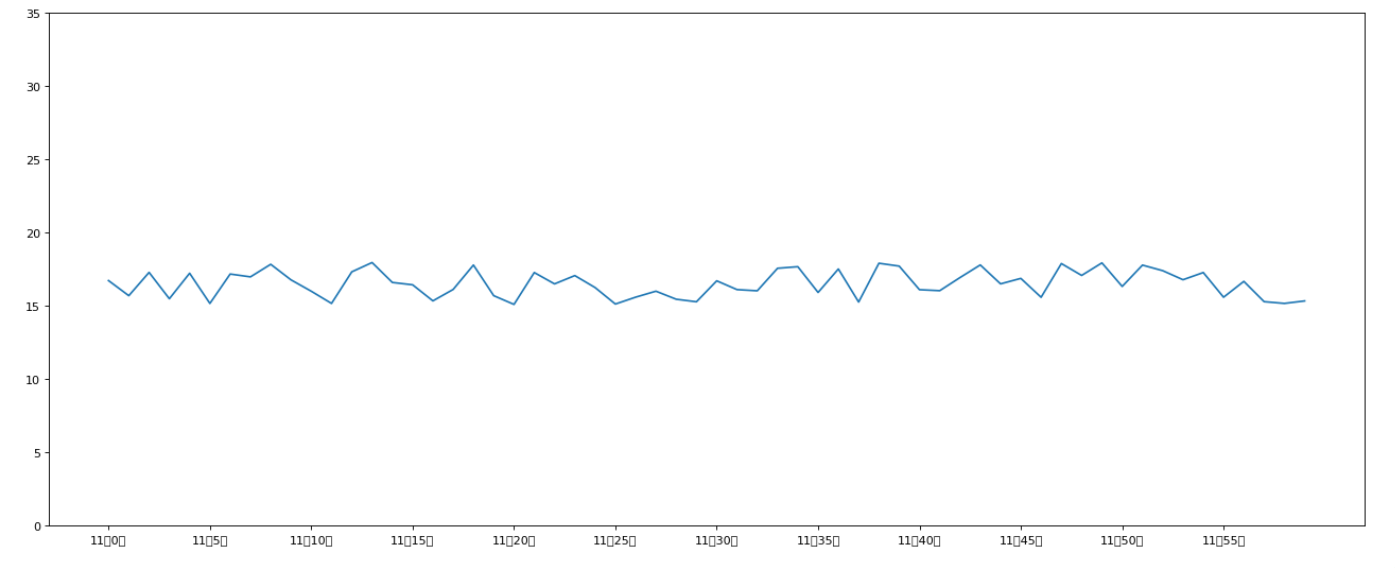3. 中文显示问题解决
可以看上面的图的x轴标签中文无法显示，可以用一下代码解决
# 加载import开头
plt.rcParams['font.sans-serif'] = ['SimHei'] # Matplotlib中设置字体-黑体，解决Matplotlib中文乱码问题 plt.rcParams['axes.unicode_minus'] = False # 解决Matplotlib坐标轴负号'-'显示为方块的问题

实际应用
import matplotlib.pyplot as plt
import random
plt.rcParams['font.sans-serif'] = ['SimHei'] # Matplotlib中设置字体-黑体，解决Matplotlib中文乱码问题 plt.rcParams['axes.unicode_minus'] = False # 解决Matplotlib坐标轴负号'-'显示为方块的问题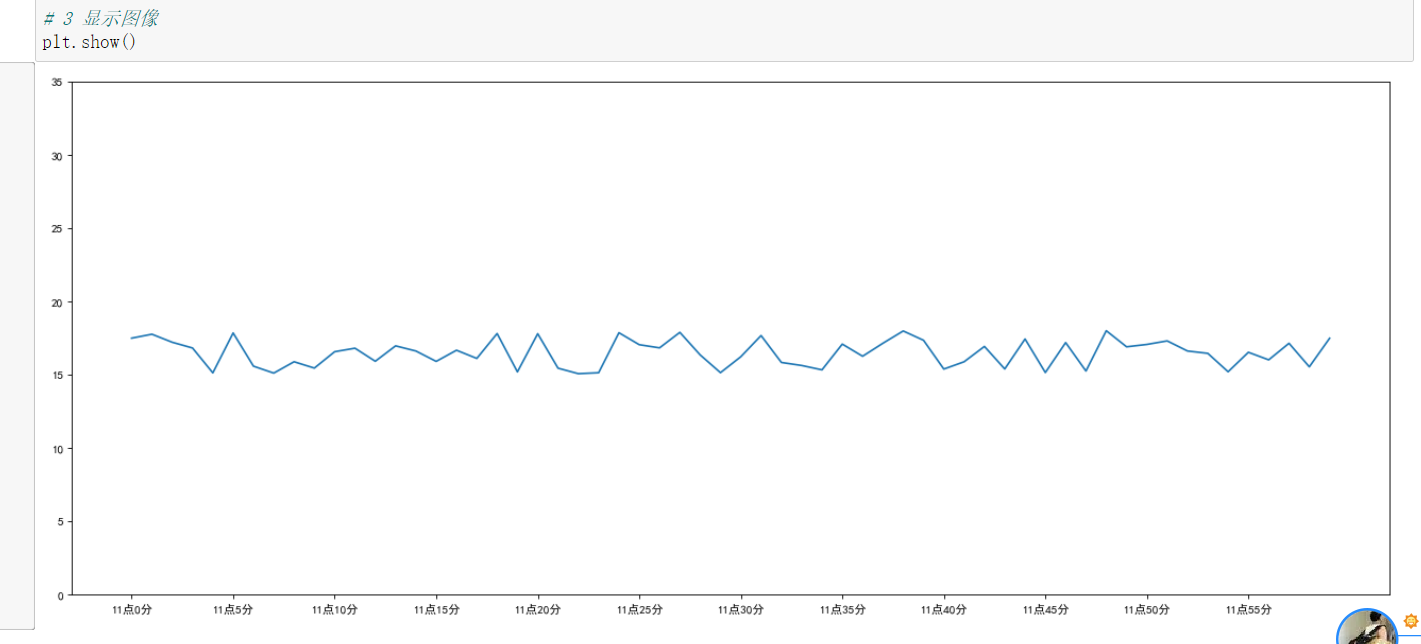4. 添加网格显示
为了更加清楚地观察图形对应的值
plt.grid(True, linestyle='--', alpha=0.5)

实际应用
# 完善原始折线图1(辅助显示层)
# 2 添加自定义x,y刻度
# 4 添加网格显示

# 准备x, y坐标的数据
x = range(60)
y_shanghai = [random.uniform(15,18) for i in x]

# 1 创建绘布
plt.figure(figsize=(20,8),dpi=80)

# 2 绘制折线图
plt.plot(x,y_shanghai)

# 3 辅助显示
# 构造x轴刻度标签
x_ticks_label = ['11点{}分'.format(i) for i in x]
# 构造y轴刻度
y_ticks_label = range(40)
# 修改x,y轴坐标的刻度显示
plt.xticks(x[::5],x_ticks_label[::5])
plt.yticks(y_ticks_label[::5])
# 添加辅助线
plt.grid(True,linestyle='--',alpha=0.5)

# 4 显示图像
plt.show()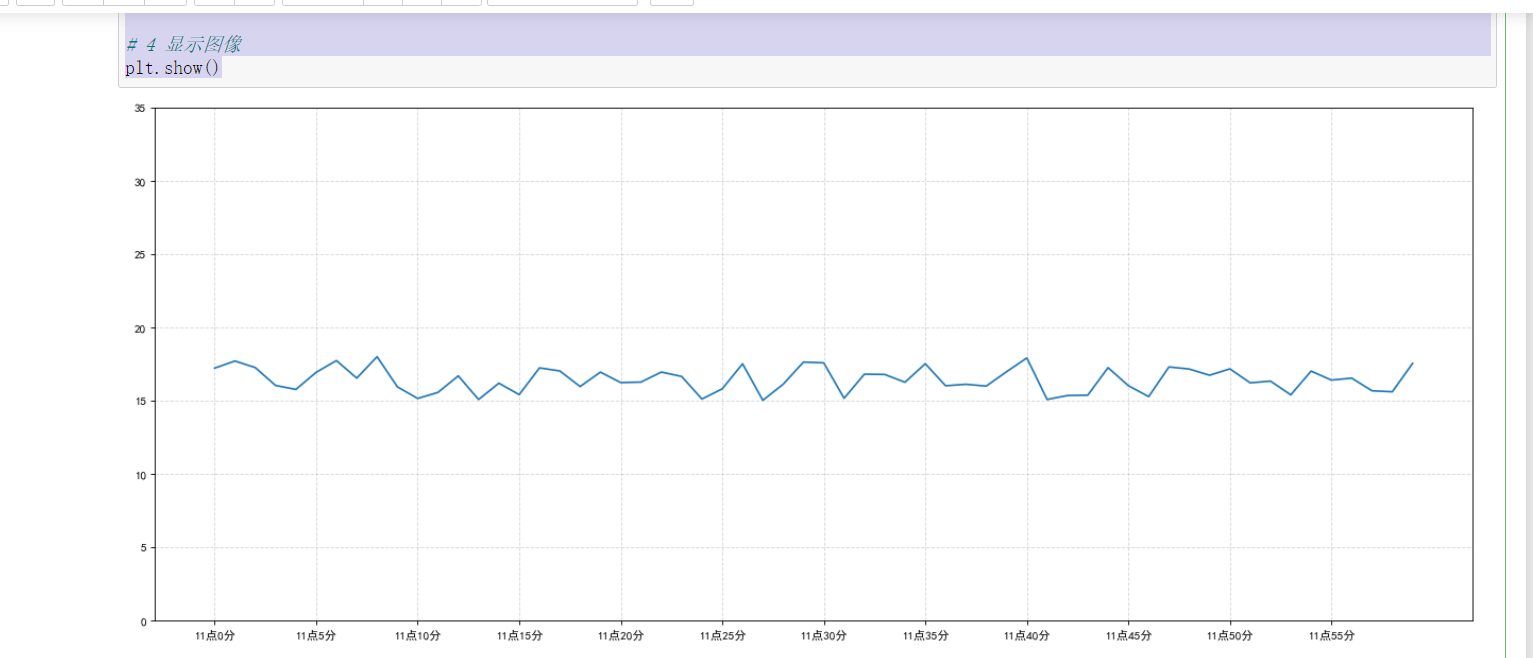5. 添加描述信息
添加x轴、y轴描述信息及标题
plt.xlabel("时间")
plt.ylabel("温度")
plt.title("中午11点0分到12点之间的温度变化图示")

实际应用
# 完善原始折线图1(辅助显示层)
# 2 添加自定义x,y刻度
# 4 添加网格显示
# 5 添加描述信息

# 准备x, y坐标的数据
x = range(60)
y_shanghai = [random.uniform(15,18) for i in x]

# 1 创建绘布
plt.figure(figsize=(20,8),dpi=80)

# 2 绘制折线图
plt.plot(x,y_shanghai)

# 3 辅助显示
# 构造x轴刻度标签
x_ticks_label = ['11点{}分'.format(i) for i in x]
# 构造y轴刻度
y_ticks_label = range(40)
# 修改x,y轴坐标的刻度显示
plt.xticks(x[::5],x_ticks_label[::5])
plt.yticks(y_ticks_label[::5])
# 添加辅助线
plt.grid(True,linestyle='--',alpha=0.5)
# 添加x,y轴描述信息及标题
plt.xlabel('时间')
plt.ylabel('温度')
plt.title('中午11点0分到12点之间的温度变化图示')

# 4 显示图像
plt.show()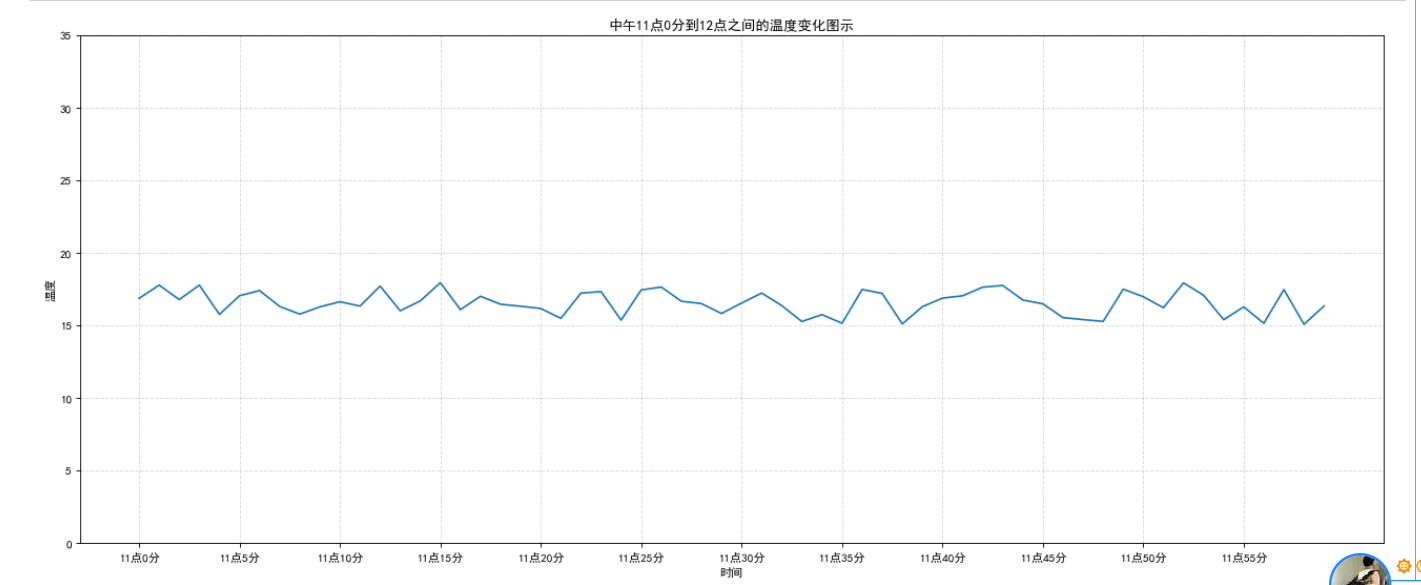完善原始折线图2(图像层)
1 多次plot
需求：再添加一个城市的温度变化收集到北京当天温度变化情况，温度在1度到3度。怎么去添加另一个在同一坐标系当中的不同图形，其实很简单只需要再次plot即可，但是需要区分线条，如下显示
# 增加北京的温度数据
y_beijing = [random.uniform(1, 3) for i in x]
# 绘制折线图
plt.plot(x, y_shanghai, label="上海")
# 使用多次plot可以画多个折线
plt.plot(x, y_beijing, color='r', linestyle='--', label="北京")
# 显示图例
plt.legend(loc="best")

我们仔细观察，用到了两个新的地方，一个是对于不同的折线展示效果，一个是添加图例,实际应用如下
# 完善原始折线图1(辅助显示层)
# 2 添加自定义x,y刻度
# 4 添加网格显示
# 5 添加描述信息

# 准备x, y坐标的数据
x = range(60)
y_shanghai = [random.uniform(15,18) for i in x]

# 1 创建绘布
plt.figure(figsize=(20,8),dpi=80)

# 2 绘制折线图
plt.plot(x,y_shanghai)

# 3 辅助显示
# 构造x轴刻度标签
x_ticks_label = ['11点{}分'.format(i) for i in x]
# 构造y轴刻度
y_ticks_label = range(40)
# 修改x,y轴坐标的刻度显示
plt.xticks(x[::5],x_ticks_label[::5])
plt.yticks(y_ticks_label[::5])
# 添加辅助线
plt.grid(True,linestyle='--',alpha=0.5)
# 添加x,y轴描述信息及标题
plt.xlabel('时间')
plt.ylabel('温度')
plt.title('中午11点0分到12点之间的温度变化图示')

# 4 显示图像
plt.show()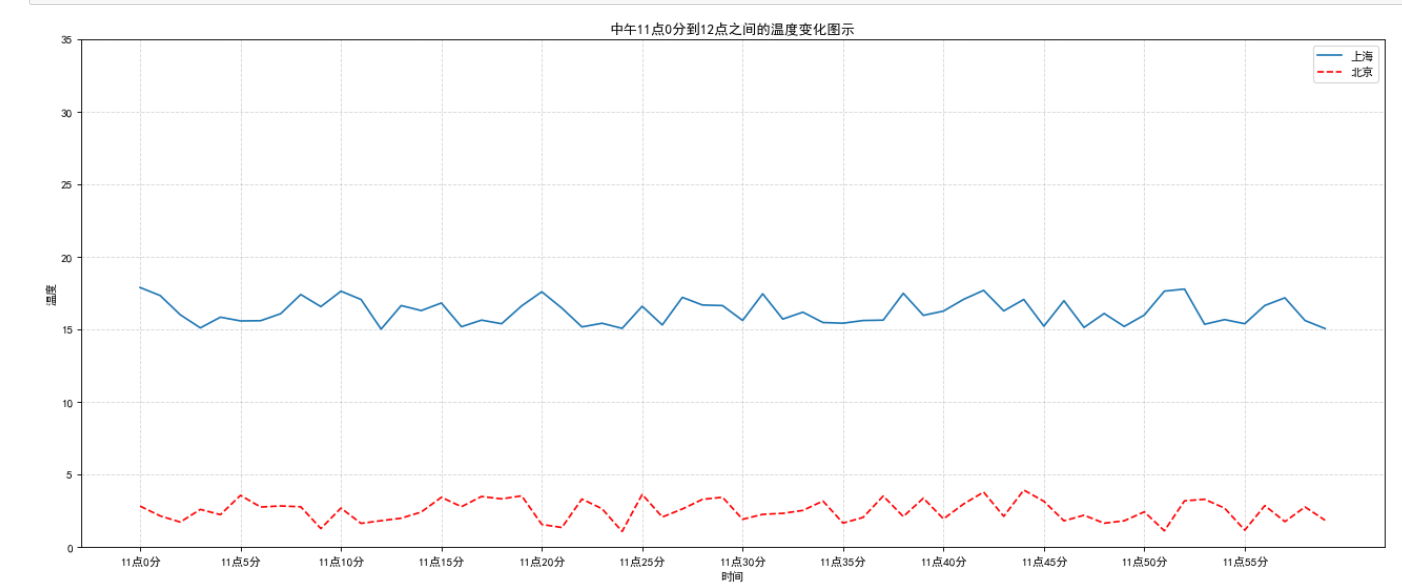2 设置图形风格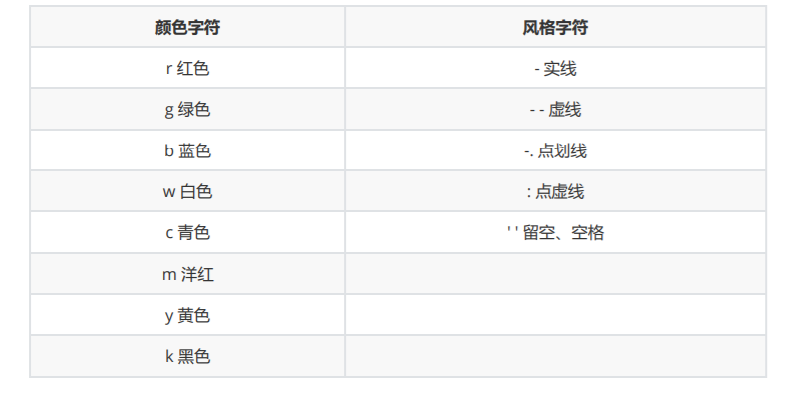显示图例
注意：如果只在plt.plot()中设置label还不能最终显示出图例，还需要通过plt.legend()将图例显示 出来。
plt.legend(loc="best")

两次完善的总结代码
import matplotlib.pyplot as plt
import random
plt.rcParams['font.sans-serif'] = ['SimHei'] # Matplotlib中设置字体-黑体，解决Matplotlib中文乱码问题 plt.rcParams['axes.unicode_minus'] = False # 解决Matplotlib坐标轴负号'-'显示为方块的问题

# 准备x, y坐标的数据
x = range(60)
y_shanghai = [random.uniform(15,18) for i in x]
# 增加北京的温度数据
y_beijing = [random.uniform(1,4) for i in x]

# 1 创建绘布
plt.figure(figsize=(20,8),dpi=80)

# 2 绘制折线图
plt.plot(x,y_shanghai,label='上海')
# 使用多次plot可以画多个折线
plt.plot(x,y_beijing,label='北京',color='r',linestyle='--')

# 3 辅助显示
# 构造x轴刻度标签
x_ticks_label = ['11点{}分'.format(i) for i in x]
# 构造y轴刻度
y_ticks_label = range(40)
# 修改x,y轴坐标的刻度显示
plt.xticks(x[::5],x_ticks_label[::5])
plt.yticks(y_ticks_label[::5])
# 添加辅助线
plt.grid(True,linestyle='--',alpha=0.5)
# 添加x,y轴描述信息及标题
plt.xlabel('时间')
plt.ylabel('温度')
plt.title('中午11点0分到12点之间的温度变化图示')
# 显示图例
plt.legend(loc='best')

# 4 显示图像
plt.show()

多个坐标系显示-plt.subplots(面向对象的画图方法)
如果我们想要将上海和北京的天气图显示在同一个图的不同坐标系当中，效果如下：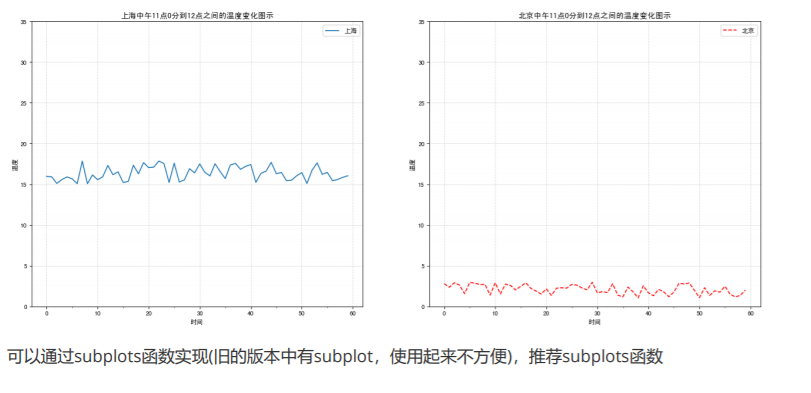可以通过subplots函数实现(旧的版本中有subplot，使用起来不方便)，推荐subplots函数
matplotlib.pyplot.subplots(nrows=1, ncols=1, **fig_kw) 创建一个带有多个axes(坐标系/绘图区)的图
关于axes子坐标系的更多方法：参考https://matplotlib.org/api/axes_api.html#matplotlib.axes.Axes
注意1：plt.函数名()相当于面向过程的画图方法，axes.set_方法名()相当于面向对象的画图方法。注意2： 使用subplot方法创建的图表不能直接使用set_xticks方法传入自定义的刻度，必须使用set_xticklabels()方法
打开上面的网址https://matplotlib.org/api/axes_api.html#matplotlib.axes.Axes F5查找到相关的方法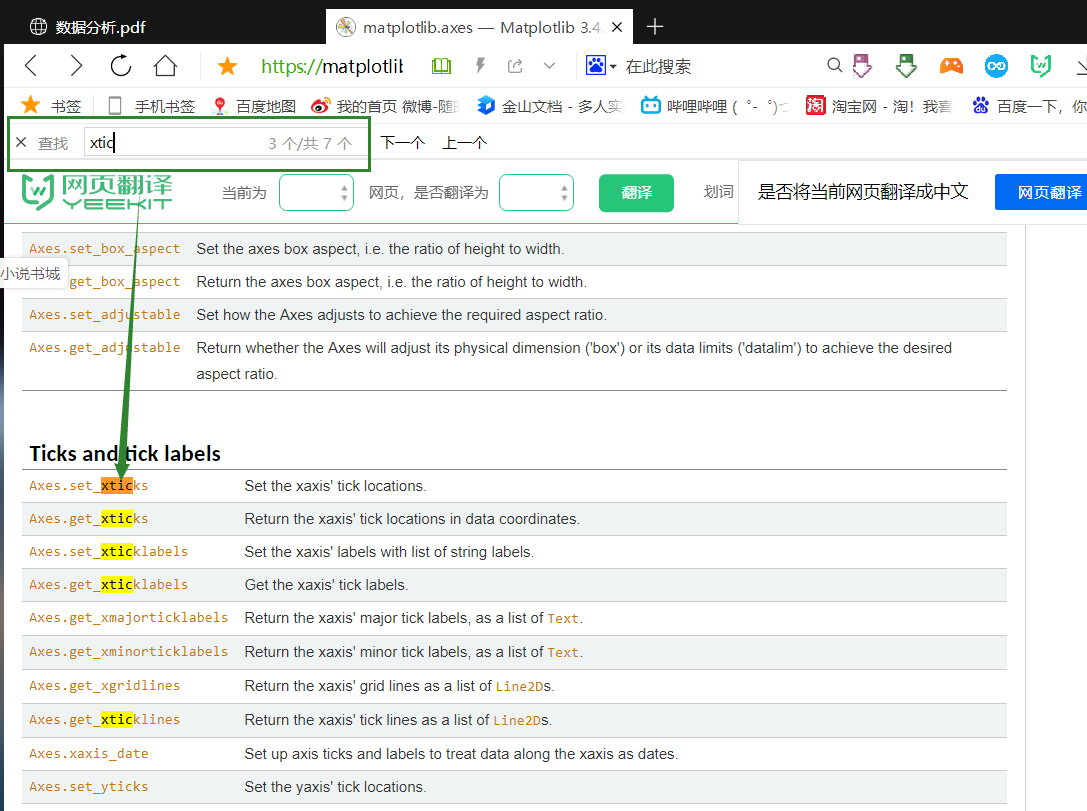axes.set_xticks()方法只能传进一个参数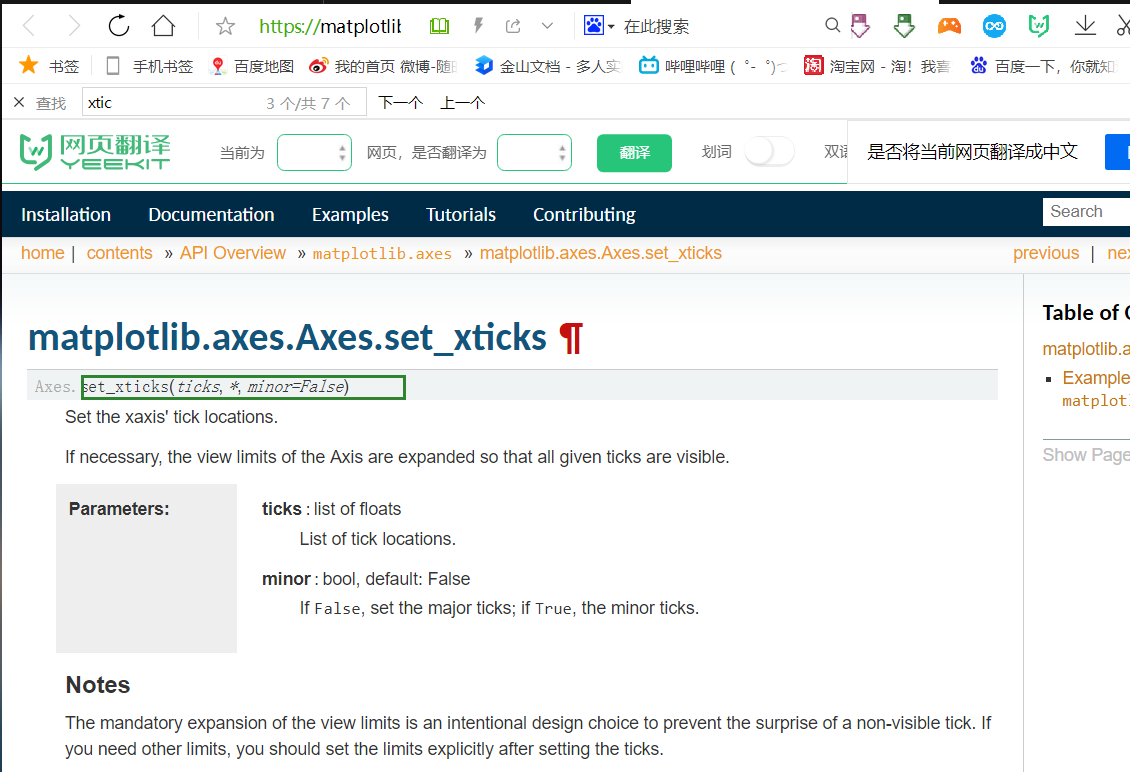因此只能使用set_xticklabels()方法传入自己自定义的标签刻度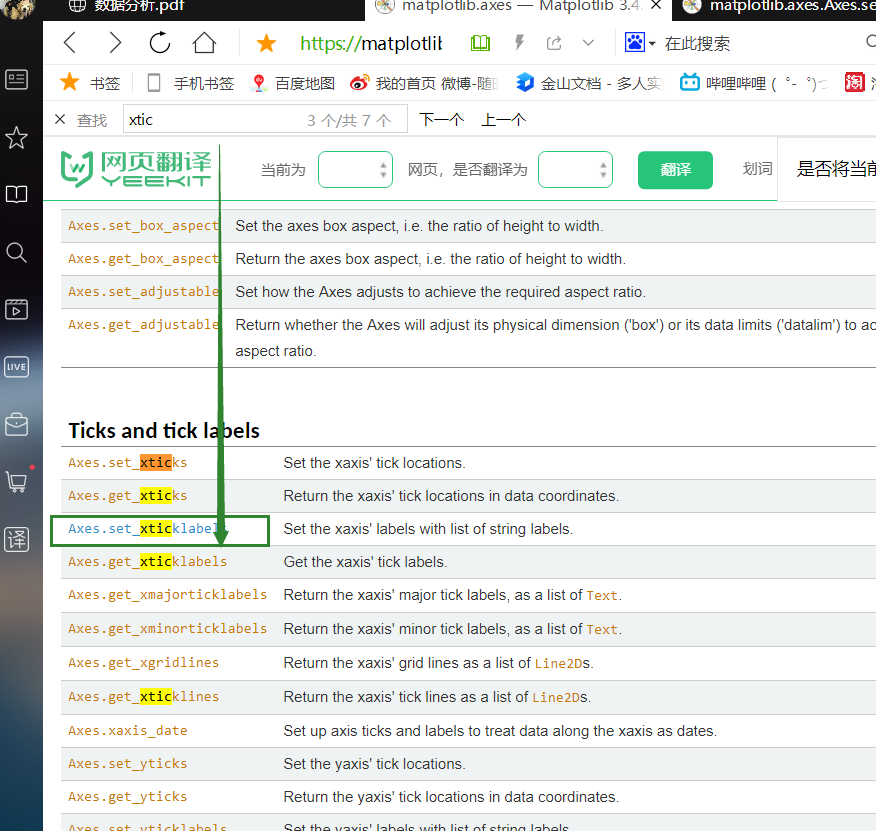# 2.2.4 多个坐标系显示-plt.subplots(面向对象的画图方法)

# 准备x, y坐标的数据
x = range(60)
y_shanghai = [random.uniform(15,18) for i in x]
# 增加北京的温度数据
y_beijing = [random.uniform(1,4) for i in x]

# 1 创建绘布
# 注意：plt.函数名()相当于面向过程的画图方法，
# axes.set_方法名()相当于面向对象的画图方法。
fig,axes = plt.subplots(nrows=1,ncols=2,figsize=(20,8),dpi=80)

# 2 绘制折线图
axes.plot(x,y_shanghai,label='上海')
# 使用多次plot可以画多个折线
axes.plot(x,y_beijing,label='北京',color='r',linestyle='--')

# 3 辅助显示
# 构造x轴刻度标签
x_ticks_label = ['11点{}分'.format(i) for i in x]
# print(x_ticks_label[::5])

# 构造y轴刻度
y_ticks_label = range(40)
# 修改x,y轴坐标的刻度显示,注意使用subplot方法创建的图表不能直接使用set_xticks方法传入自定义的刻度，必须使用set_xticklabels()方法
axes.set_xticks(x[::5])
axes.set_xticklabels(x_ticks_label[::5])
axes.set_yticks(y_ticks_label[::5])
axes.set_xticks(x[::5])
axes.set_xticklabels(x_ticks_label[::5])
axes.set_yticks(y_ticks_label[::5])
# 添加辅助线
axes.grid(True,linestyle='--',alpha=0.5)
axes.grid(True,linestyle='--',alpha=0.5)
# 添加x,y轴描述信息及标题
axes.set_xlabel('时间')
axes.set_ylabel('温度')
axes.set_title('上海中午11点0分到12点之间的温度变化图示')
axes.set_xlabel('时间')
axes.set_ylabel('温度')
axes.set_title('北京中午11点0分到12点之间的温度变化图示')
# 显示图例
axes.legend()
axes.legend()

# 4 显示图像
plt.show()小结
开头的这几个目标应用全都很重要
知道如何解决中文显示问题知道matplotlib的图结构应用figure实现创建绘图区域大小应用plot实现折线图的绘制应用title,xlabel,ylabel实现标题以及x,y轴名设置应用xticks,yticks实现axes的刻度设置和标注应用savefig实现图形的本地保存应用grid实现显示网格应用axis实现图像形状修改应用legend实现图形标注信息显示应用plt.subplots实现多坐标系的创建知道如何设置多个axes的标题、刻度
展开全文python jupyter
• 显示图像需要配合PIL库（pythonpillow库） 导入matplotlib.pyplot库 导入matplotlib.patches库 # picdir是图片的路径 # 直接采用Image.open(picdir, 'r')的方法，没有关闭文件的方法，易导致内存泄漏 # 因此采用...
matplotlib显示图像
显示图像需要配合PIL库（python中pillow库）导入matplotlib.pyplot库导入matplotlib.patches库
# picdir是图片的路径
# 直接采用Image.open(picdir, 'r')的方法，没有关闭文件的方法，易导致内存泄漏
# 因此采用以下方法，通过二进制文件句柄的方式开和关闭

pf = open(picdir, 'rb')
img = Image.open(pf)
pf.close()

matplotlib在图像上添加标记框
# 注意这个方法是调用Patches在原图上绘制一个片
# facecolor设置为'none'可以使矩形框不填充颜色
# 坐标取的是左上角和宽度、高度
# patches添加的位置是轴句柄，而不是fig句柄

fig, ax1 = plt.subplots(1)
p = plt.imshow(img)
upleft = (x0, y0)
width = x1-x0
height = y1-y0
rect = patches.Rectangle(upleft, width, height, linewidth=0.3, edgecolor='r', facecolor='none')

matplotlib保存图像并去掉周边的空白
# 通过plt.gcf()选取前景，这样才能保证只绘制图像本身
# bbox_inches设置为'tight'
# 提前将轴隐去

ax1.axis('off')
fig = plt.gcf()

matplotlib方法存在内存泄漏问题
imshow()这个方法总是在创建新的对象该对象没有办法自动关闭对大量图像进行循环操作时尤其需要注意这问题，内存是无法释放的针对这个问题，建议需要大量操作图像的用pillow自带的ImageDraw模块或者用cv模块来实现，matplotlib还是适用于少量的曲线绘制，数据呈现等。
展开全文深度学习
• 欢迎关注”生信修炼手册”!当我们想要一个坐标系包含另外一个完整的图像时，就需要用到子图相关的技术，在matplotlib中，提供了以下两种实现方式1.画中画通过原本axes插入...

欢迎关注”生信修炼手册”!
当我们想要在一个坐标系中包含另外一个完整的图像时，就需要用到子图相关的技术，在matplotlib中，提供了以下两种实现方式
1. 画中画
通过在原本axes中插入一个新的axes, 来实现画中画的目的，代码如下
>>> fig,ax = plt.subplots()
>>> ax.scatter(x= np.random.randn(10), y=np.random.randn(10),s=40 * np.arange(10),c=np.random.randn(10))
>>> ax1 = ax.inset_axes([0.6, 0.5, 0.3, 0.45])
>>> ax1.scatter(x= np.random.randn(10), y=np.random.randn(10),s=40 * np.arange(10),c=np.random.randn(10))
>>> plt.show()
输出结果如下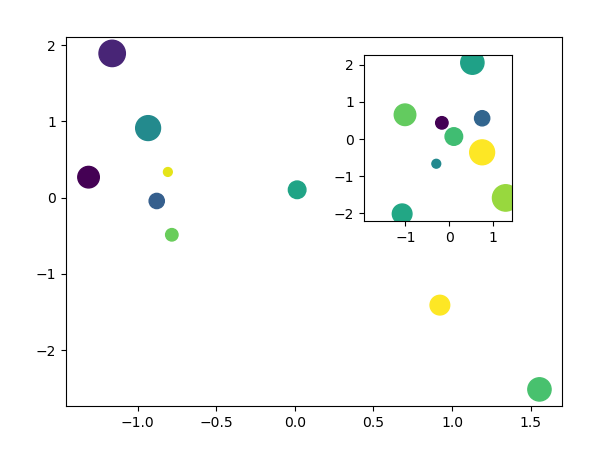这种方式本质上两个axes是相互独立的，所以可以实现任意两个图像的叠加，非常的灵活。
2. 缩放图
顾名思义，对图中的局部区域进行缩放，属于画中画的一种特殊情况，在matplotlib中，针对这一场景，专门提供了indicate_inset_zoom，来实现缩放图，用法如下
>>> fig,ax = plt.subplots()
>>> seed = 123456
>>> np.random.seed(seed)
>>> ax.scatter(x= np.random.randn(10), y=np.random.randn(10),s=40 * np.arange(10),c=np.random.randn(10))
>>> ax1 = ax.inset_axes([0.6, 0.5, 0.32, 0.45])
>>> np.random.seed(seed)
>>> ax1.scatter(x= np.random.randn(10), y=np.random.randn(10),s=40 * np.arange(10),c=np.random.randn(10))
>>> ax1.set_xlim(-1.5, -0.8)
>>> ax1.set_ylim(-0.8, -0.3)
>>> ax.indicate_inset_zoom(ax1)
>>> plt.show()
输出结果如下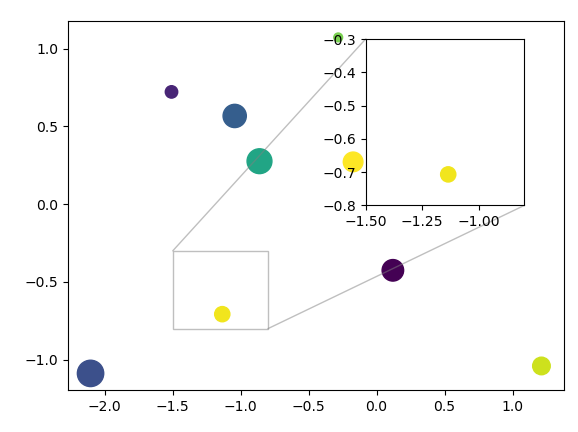在缩放图中，会将原本的区域和缩放之后的区域用线条连接起来，这是缩放图独有的特征。
·end·
—如果喜欢，快分享给你的朋友们吧—
原创不易，欢迎收藏，点赞，转发！生信知识浩瀚如海，在生信学习的道路上，让我们一起并肩作战！
本公众号深耕耘生信领域多年，具有丰富的数据分析经验，致力于提供真正有价值的数据分析服务，擅长个性化分析，欢迎有需要的老师和同学前来咨询。
更多精彩
KEGG数据库，除了pathway你还知道哪些全网最完整的circos中文教程DNA甲基化数据分析专题突变检测数据分析专题mRNA数据分析专题lncRNA数据分析专题circRNA数据分析专题miRNA数据分析专题单细胞转录组数据分析专题chip_seq数据分析专题Hi-C数据分析专题HLA数据分析专题TCGA肿瘤数据分析专题基因组组装数据分析专题CNV数据分析专题GWAS数据分析专题2018年推文合集2019年推文合集
写在最后
转发本文至朋友圈，后台私信截图即可加入生信交流群，和小伙伴一起学习交流。
扫描下方二维码，关注我们，解锁更多精彩内容！一个只分享干货的
生信公众号

展开全文微软 xhtml nagios 数据分析
• 刚开始接触maplotlib，安装之后第一...import matplotlib.pyplot as plt plt.axis([0,5,0,20]) plt.title('my first plot') plt.plot([1,2,3,4],[1,4,9,16],'ro') 这里是简单的生成图像的操作，运行之后发现图像并...
• 首先补充一下：两种体系7种颜色rgbymck（红，绿，蓝，黄，品红，青，黑）科研的过程，坐标系的XY不一定就是等尺度的。例如声波对Y轴取对数。肆意我们也必须知道这种坐标系如何出来的。有3个函数可以实现...
• ## 用matplotlib画线

千次阅读 2018-03-31 08:58:22
就拿sin、cossin、cos\sin 、\cos来...import matplotlib.pyplot as plt import numpy import math #创建x数组，区间是[0,4π]，步长为0.1 x = numpy.arange(0, 4 * math.pi, 0.1) def sinFunction(x): sin = [ma...曲线 sin cos
• 介绍Matplotlib是Python使用最广泛的数据可视化库之一。Matplotlib的受欢迎程度大部分来自其自定义选项。您可以调整其对象层次结构的几乎任何元素。本教程，我们将研...python 数据可视化 css js numpy
• 1.矩形 　这个费了我半天劲，不知怎么就可以了。 　复制来自：https://www.cnblogs.com/ymjyqsx/p/7390288.html　 import matplotlib.pyplot as plt fig = plt.figure()ax = fig.add_subplot(111) #创建子图,...
• 怎样出如下所示的3D图像： 代码如下： import matplotlib.pyplot as plt from numpy import * from mpl_toolkits.mplot3d import Axes3D #设置坐标轴 fig=plt.figure() #定义一个窗口 ax=Axes3D(fig) #窗口...
• 可以将画布的图像显示到UI，相当于pyqt5的一个控件，后面的绘图就建立这个画布上，然后把这个画布当中pyqt5的控件添加到pyqt5的UI上，其次要导入matplotlib.figure的Figure ，这里要注意的是matplotlib.figure的...
• ## Pycharm Matplotlib图像不显示问题

万次阅读 多人点赞 2017-11-17 11:40:50
学习Matplotlib的时候，Pycharm运行的时候不会弹出图像。 首先你运行之后最小化pycharm，看看是不是已经出来了，只是没有自己弹到最顶层。   代码如下：   import numpy as np import matplotlib....
• dashed_dot点划线 ‘:’ dotted虚线 ‘None’ draw nothing ’ ’ draw nothing ‘’ draw nothing 点标记 描述 “o” circle圆圈 “v” ...python
• matplotlib 是 python 最著名的绘图库，它...因此如果你需要绘制某种类型的图，只需要这个页面浏览/复制/粘贴一下，基本上都能搞定。这是要实现的效果图，利用 Matplotlib 绘制函数 y = x^2^ 的图像。image代...
• 出两个函数y1 = x1，y2 = x2 ** 0.5范围{ (x1,y1) | 1 <= x <= 2, x1 ** 0.5 <= y <= x1 }， { (x2,y2) | 2 <= x <= 4,2 <= y <= x1 ** 0.5 } 内的图像，代码如下： import ...
• 利用python中matplotlib库，实现绘制牛顿插值、拉格朗日插值、三次样条插值函数图像。 随机在图像上产生5个随机点，用三种插值方式求函数，并用python中matplotlib库绘制在图像
• 1.绘制折线图,并给出解决中文无法...import matplotlib.pyplot as plt import matplotlib#方法一,改变全局字体 matplotlib.rcParams['font.family']='SimHei' matplotlib.rcParams['font.size'] = 12 plt.subplot(211)
• 转载请注明：虚幻私塾 » matplotlib figure 图像 简单的线条 matplotlib 的 figure 就是一个 单独的 figure 小窗口, 小窗口里面还可以有更多的小图片. 使用import导入模块matplotlib.pyplot，并简写成plt 使用...
• 主要介绍了Python使用matplotlib绘制正弦和余弦曲线的方法,结合实例形式分析了Python调用matplotlib库进行图形绘制想具体操作技巧,需要的朋友可以参考下
• 在上一篇文章当中我们介绍了matplotlib当中subplot的概念以及用法，今天我们将会来介绍matplotlib绘图的一些具体的设置，可以让我们出来的图像更加丰富，表现力也更强。 颜色 我们之前绘制的图像都是蓝色的，这...python 机器学习
• 首先如何python安装matplotlib库呢？ cmd使用命令 pip install matplotlib 任务目标： 代码实例： import matplotlib.pyplot as plt #加载matplotlib库的pyplot模块并起别名叫plt import numpy as np #再...python
• 1.绘制图形,图像过程,可以借助绘制时间的控制,逐步显示绘制内容,进而达到图形, 图像动态显示的效果 用plot()和scatter()绘制正弦波和散点图，其中散点图是动态沿着正弦波绘制.实现代码如下(我的测试环境是IDIE，...python
• Matplotlib 是一个非常强大的 Python 画图工具，通过绘制...在matplotlib的层级结构，最高层是由matplotlib.pyplot模块提供的“状态机环境”（state-machine environment），通过它来为当前维度绘制图像元素，例如...
• 实际上前面我们就已经用到了图像的绘制，如： io.imshow(img) 这一行代码的实质是利用matplotlib包对图片进行绘制，绘制成功后，返回一个matplotlib类型的数据。因此，我们也可以这样写： import matplotlib....
• import matplotlib.pyplot as plt 准备数据 x = [1, 2, 3, 4, 5, 6, 7, 8, 9, 10, 11, 12] y = [3145, 222, 2342, 9002, 2031, 3213, 4535, 4332, 2134, 2000, 1672, 1213] 二、修饰代码 描述：修饰图像信息，如...
• 需求： 实现一张同时出现的 sin 图像与 cos 图像 sin图像用实线表示，cos图像用...import matplotlib.pyplot as plt import numpy as np plt.rcParams['font.sans-serif'] = ['SimHei'] # 用来正常显示中文标签 ...python
• 本教程将使用matplotlib的命令式绘图接口pyplot。这个界面保持全局状态，并且对于快速和容易地尝试各种绘图设置是非常有用的。另一种是面向对象的接口，它也是非常强大的，一般更适合大型应用程序的开发。如果您想...Python 可视化
• 导入matplotlib: import matplotlib.pyplot as plt 使用内部函数plot(）来绘制图线 plt.plot(Close['2020']) 执行完代码图像未显示出来时，还需要用到show()方法。 plt.show() 默认方式过于简单而漏掉了很多信息...python 可视化 数据分析 数据可视化
• 目录 1 灰度直方图简介 1.1 灰度直方图概念 1.2 灰度直方图作用 1.3 绘制的直方图 1.4 归一化直方图 ...灰度直方图（histogram）是灰度级的函数，描述的是图像中每种灰度级像素的个数，反映...Python OpenCV...